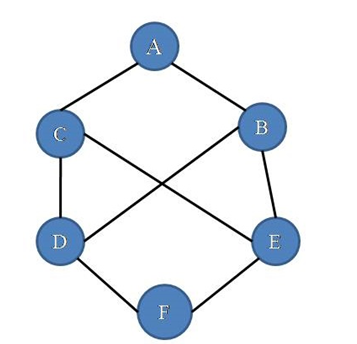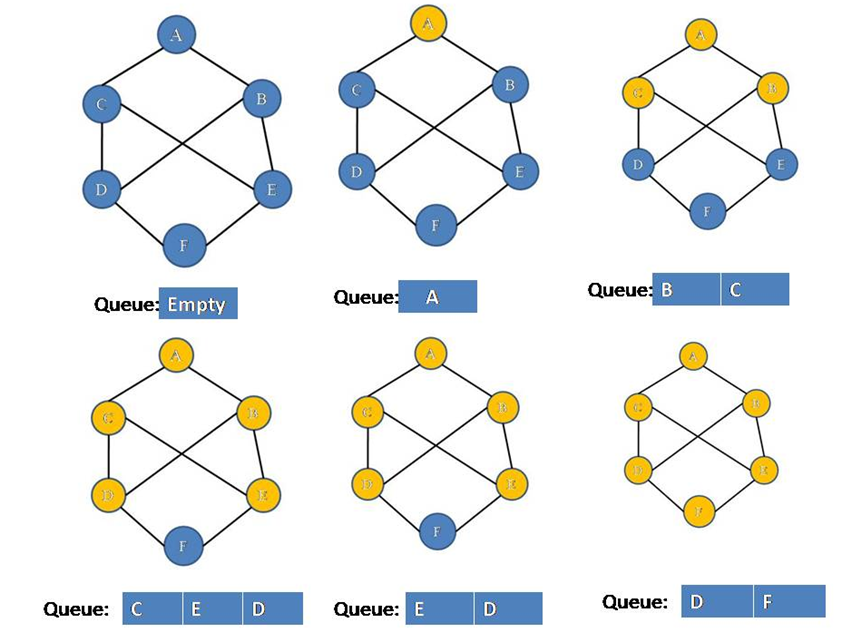# Depth First Search (DFS) of a Graph

In this article, we are going to see graph traversal method (DFS) with C++ implementation.
Submitted by Souvik Saha, on March 19, 2019

What you will learn?

How to implement Depth first search of a graph?

Depth First Search is a depthwise vertex traversal process. Like a tree all the graphs have vertex but graphs have cycle so in searching to avoid the coming of the same vertex we prefer DFS.

Algorithm:

To implement the DFS we use stack and array data structure.

There are two cases in the algorithm:

1. Whenever we visit a vertex we mark it visited and push its adjacent non-visited vertices into the stack and pop the current vertex from the stack.
2. If all the adjacent vertices are visited then only pop the current vertex from the stack.

Consider this graph,Our algorithm performs like following for the above graph:Hence all the vertices are visited then only pop operation is performed and stack will be empty at last.

C++ Implementation:

```#include <iostream>
#include <bits/stdc++.h>
#include <list>
using namespace std;

void DFS(list<int>*,int);

//	Make a pair between vertex x and vertex y
ls[x].push_back(y);
ls[y].push_back(x);
return;
}

//Depth First Search of a Graph
void DFS(list<int>*ls,int num,int x){
bool *visit= new bool[num];
for(int i=0;i<num;i++){
visit[i]=false;
}
stack<int> st;
st.push(x);
while(!st.empty()){
int s=st.top();
st.pop();
if(!visit[s]){
cout<<s<< " ";
visit[s]=true;
list<int>::iterator it;
for(it=ls[s].begin();it!=ls[s].end();it++){
st.push(*it);
}
}
}
}

void print(list<int> *ls,int num){
list<int>::iterator it;
for(int i=0;i<6;i++){
cout<<i<<"-->";
for(it=ls[i].begin();it!=ls[i].end();it++){
cout<<*it<<"-->";
}
cout<<endl;
}
}

int main(){
int num=6;

cout<<"Enter the no. of vertices : 6\n";
list<int> *ls=new list<int>[num];

print(ls,6);
cout<<"DFS"<<endl;
DFS(ls,6,0);

return 0;
}
```

Output

```Enter the no. of vertices : 6
0-->2-->3
1-->4
2-->0-->3-->5
3-->2-->4-->0
4-->3-->5-->1
5-->4-->2
DFS:
0 3 4 1 5 2

```